JMSLTM Numerical Library 7.2.0
com.imsl.io

• All Implemented Interfaces:
Serializable

extends Object
implements Serializable
Reads a linear programming problem from an MPS file.

An MPS file defines a linear or quadratic programming problem. Linear programming problems read using this class are assumed to be of the form: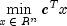subject to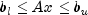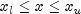where c is the objective coefficient vector, A is the coefficient matrix, and the vectors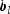,,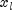, and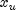are the lower and upper bounds on the constraints and the variables, respectively.

The following table helps map this notation into the use of MPSReader.

 C Objective A Constraint matrixLower Range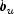Upper RangeLower BoundUpper Bound

If the MPS file specifies an equality constraint or bound, the corresponding lower and upper values will be exactly equal.

The problem formulation assumes that the constraints and bounds are two-sided. If a particular constraint or bound has no lower limit, then the corresponding entry in the structure is set to negative machine infinity. If the upper limit is missing, then the corresponding entry in the structure is set to positive machine infinity.

MPS File Format

There is some variability in the MPS format. This section describes the MPS format accepted by this reader.

An MPS file consists of a number of sections. Each section begins with a name in column 1. With the exception of the NAME section, the rest of this line is ignored. Lines with a '*' or '\$' in column 1 are considered comment lines and are ignored.

The body of each section consists of lines divided into fields, as follows:

 Field Number Columns Content 1 2-3 Indicator 2 5-12 Name 3 15-22 Name 4 25-36 Value 5 40-47 Name 6 50-61 Value

The format limits MPS names to 8 characters and values to 12 characters. The names in fields 2, 3 and 5 are case sensitive. Leading and trailing blanks are ignored, but internal spaces are significant.

The sections in an MPS file are as follows:

NAME

ROWS

COLUMNS

RHS

RANGES (optional)

BOUNDS (optional)

ENDATA

Sections must occur in the above order.

MPS keywords, section names and indicator values, are case insensitive. Row, column and set names are case sensitive.

NAME Section

The NAME section contains the single line. A problem name can occur anywhere on the line after NAME and before columns 62. The problem name is truncated to 8 characters.

ROWS Section

The ROWS section defines the name and type for each row. Field 1 contains the row type and field 2 contains the row name. Row type values are not case sensitive. Row names are case sensitive. The following row types are allowed:

 Row Type Meaning E Equality constraint L Less than or equal constraint G Greater than or equal constraint N Objective of a free row

COLUMNS Section

The COLUMNS section defines the nonzero entries in the objective and the constraint matrix. The row names here must have been defined in the ROWS section.

 Field Contents 2 Column name 3 Row name 4 Value for the entry whose row and column are given by fields 2 and 3 5 Row name 6 Value for the entry whose row and column are given by fields 2 and 5

Note: Fields 5 and 6 are optional.

The COLUMNS section can also contain markers. These are indicated by the name 'MARKER' (with the quotes) in field 3 and the marker type in field 4 or 5.

Marker type 'INTORG' (with the quotes) begins an integer group. The marker type 'INTEND' (with the quotes) ends this group. The variables corresponding to the columns defined within this group are required to be integer.

RHS Section

The RHS section defines the right-hand side of the constraints. An MPS file can contain more than one RHS set, distinguished by the RHS set name. The row names here must be defined in the ROWS section.

 Field Contents 2 RHS name 3 Row name 4 Value for the entry whose row and column are given by fields 2 and 3 5 Row name 6 Value for the entry whose row and column are given by fields 2 and 5

Note: Fields 5 and 6 are optional.

RANGES Section

The optional RANGES section defines two-sided constraints. An MPS file can contain more than one range set, distinguished by the range set name. The row names here must have been defined in the ROWS section.

 Field Contents 2 Range set name 3 Row name 4 Value for the entry whose row and column are given by fields 2 and 3 5 Row name 6 Value for the entry whose row and column are given by fields 2 and 5

Note: Fields 5 and 6 are optional.

Ranges change one-sided constraints, defined in the RHS section, into two-sided constraints. The two-sided constraint for row i depends on the range value,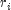, defined in this section. The right-hand side value,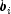, is defined in the RHS section. The two-sided constraints for row i are given in the following table:

 Row Type Lower Constraint Upper Constraint G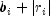L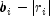E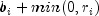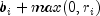BOUNDS Section

The optional BOUNDS section defines bounds on the variables. By default, the bounds are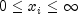. The bounds can also be used to indicate that a variable must be an integer.

More than one bound can be set for a single variable. For example, to set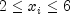use a LO bound with value 2 to set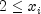and an UP bound with value 6 to add the condition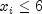.

An MPS file can contain more than one bounds set, distinguished by the bound set name.

 Field Contents 1 Bounds type 2 Bounds set name 3 Column name 4 Value for the entry whose set and column are given by fields 2 and 3 5 Column name 6 Value for the entry whose set and column are given by fields 2 and 5

Note: Fields 5 and 6 are optional.

The bound types are as follows. Hereare the bound values defined in this section, the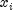are the variables, and I is the set of integers.

 Bound Type Definintion Formula LO Lower bound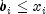UP Upper bound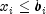FX Fixed Variable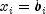FR Free variable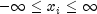MI Lower bound is minus infinity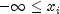PL Upper bound is positive infinity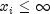BV Binary variable (variable must be 0 or 1)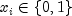UI Upper bound and integerand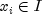LI Lower bound and integerandSC Semicontinuous 0 orThe bound type names are not case sensitive.

If the bound type is UP or UI andthen the lower bound is set to.

ENDATA Section

The ENDATA section ends the MPS file.

Example, Serialized Form
• Field Detail

• BINARY_VARIABLE

public static final int BINARY_VARIABLE
Variable must be either 0 or 1.
Constant Field Values
• CONTINUOUS_VARIABLE

public static final int CONTINUOUS_VARIABLE
Variable is a real number.
Constant Field Values
• INTEGER_VARIABLE

public static final int INTEGER_VARIABLE
Variable must be an integer.
Constant Field Values

• Method Detail

• getLowerBound

public double getLowerBound(int iVariable)
Returns the lower bound for a variable.
Parameters:
iVariable - is the number of the variable.
• getLowerRange

public double getLowerRange(int iRow)
Returns the lower range value for a constraint equation.
Parameters:
iRow - is the row number of the equation.
• getName

public String getName()
Returns the name of the MPS problem. This is the value of the NAME field.
• getNameBounds

public String getNameBounds()
Returns the name of the BOUNDS set. An MPS file can contain multiple sets of BOUNDS, but only one is retained by this reader. Returns null if there is no BOUNDS set.
• getNameObjective

public String getNameObjective()
Returns the name of the free row containing the objective.
• getNameRanges

public String getNameRanges()
Returns the name of the RANGES set. An MPS file can contain multiple sets of RANGES, but only one is retained by this reader. Returns null if there is no RANGES set.
• getNameRHS

public String getNameRHS()
Returns the name of the RHS section.
• getNameRow

public String getNameRow(int iRow)
Returns the name of a contraint row.
• getNumberOfBinaryConstraints

public int getNumberOfBinaryConstraints()
Returns the number of binary constraints. An binary constraint is the requirment that a variable be either 0 or 1. Binary constraints are also integer contraints.
• getNumberOfColumns

public int getNumberOfColumns()
Returns the number of columns in the constraint matrix.
• getNumberOfIntegerConstraints

public int getNumberOfIntegerConstraints()
Returns the number of integer constraints. An integer constraint is the requirment that a variable be an integer.
• getNumberOfNonZeros

public int getNumberOfNonZeros()
Returns the number of nonzeros in the constraint matrix.
• getNumberOfRows

public int getNumberOfRows()
Returns the number of rows in the constraint matrix.
• getObjective

Returns the objective as a Row.
• getObjectiveCoefficients

public double[] getObjectiveCoefficients()
Returns the coefficents of the objective row.
• getRow

Returns a row of the constraint matrix or a free row.
Parameters:
iRow - is the number of the row.
• getRowCoefficients

public double[] getRowCoefficients(int iRow)
Returns the coefficents of a row.
Parameters:
iRow - is the number of the row.
• getTypeVariable

public int getTypeVariable(int iVariable)
Returns the type of a variable. The variable types are CONTINUOUS_VARIABLE, BINARY_VARIABLE or INTEGER_VARIABLE.
Parameters:
iVariable - is the number of the variable.
• getUpperBound

public double getUpperBound(int iVariable)
Returns the upper bound for a variable.
Parameters:
iVariable - is the number of the variable.
• getUpperRange

public double getUpperRange(int iRow)
Returns the upper range value for a constraint equation.
Parameters:
iRow - is the row number of the equation.
• setNameBounds

public void setNameBounds(String nameBounds)
Sets the name of the BOUNDS set to be used. An MPS file can contain multiple sets of BOUNDS, but only one is retained by this reader. If not set name is set, then the first set in the file is used.
• setNameObjective

public void setNameObjective(String nameObjective)
Sets the name of the free row containing the objective. An MPS file can contain free rows, but only one is retained by this reader as the objective. If not set name is set, then the first free row in the file is used as the objective.
• setNameRanges

public void setNameRanges(String nameRanges)
Sets the name of the RANGES set to be used. An MPS file can contain multiple sets of RANGES, but only one is retained by this reader. If not set name is set, then the first set in the file is used.
• setNameRHS

public void setNameRHS(String nameRHS)
Sets the name of the RHS set to be used. An MPS file can contain multiple sets of RHS values, but only one is retained by this reader. If not set name is set, then the first set in the file is used.
JMSLTM Numerical Library 7.2.0